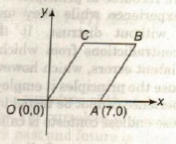Monday, September 27, 2021
Home > Question and Answer > Coordinate Geometry Question And Answer Set 1

# Coordinate Geometry Question And Answer Set 1# Coordinate Geometry Question and Answer Set 1

Hi students, welcome to Amans Maths Blogs (AMB). On this post, you will get the Coordinate Geometry Question and Answer Set 1 is the collection of some important questions. Practice these questions for SSC CGL CHSL CAT NTSE exams etc. It will help you to practice the questions on the topics of maths as coordinate geometry based questions of algebra.

Coordinate Geometry Question and Answer Set 1: Ques No 1

The coordinates of the point which will divide the line joining the points (3, 5) and (11, 8) externally in the ratio 5 : 2 is

Options:

A. (5/3, 1/3)

B. (3/49, 1/10)

C. (49/3, 10)

D. None of these

Coordinate Geometry Question and Answer Set 1: Ques No 2

In a triangle ABC, if A = (-2, 3), B = (6, 7) and C = (4, 1), then the co-ordinates of the point of intersection of the medians of triangle ABC is

Options:

A. (5/3, 1/3)

B. (3/8, 3/11)

C. (8/3, 11/3)

D. (5/3, 17/3)

Coordinate Geometry Question and Answer Set 1: Ques No 3

The three vertices of a triangle are (41,0) , (0,41) and (0,0), each vertex being represented by its (X,Y) coordinates. The number of points with integer coordinates inside the triangle (excluding all the points on the boundary) is

Options:

A. 312

B. 780

C. 835

D. 428

Coordinate Geometry Question and Answer Set 1: Ques No 4

The three vertices of a triangle are (5k, 2k), (7k, 0) and (5k, 0), each vertex being represented by its (X,Y) coordinates (k is a positive integer). If the area of the triangle is 72 sq. unit, then the value of k is

Options:

A. 6

B. 3

C. 9

D. 12

Coordinate Geometry Question and Answer Set 1: Ques No 5

The vertices of a quadrilateral ABCD are A(0, 0), B(4, 5), C(9, 9) and D(5, 4). The shape of the ABCD is

Options:

A. Square

B. Rectangle but not Square

C. Parallelogram but not Rhombus

D. Rhombus

Coordinate Geometry Question and Answer Set 1: Ques No 6

In the figure, OABC is a parallelogram. The area of parallelogram is 21 sq unit and the point C lies on line x = 3. The coordinate of B isOptions:

A. (3, 10)

B. (10, 3)

C. (10, 10)

D. (8, 3)

Get Online Maths Tuition by AMBiPi for Class 8 to 12